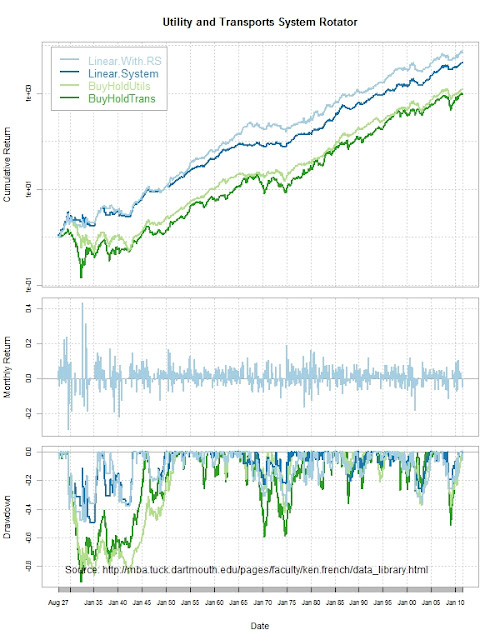Want to share your content on R-bloggers? click here if you have a blog, or here if you don't.

THIS IS NOT INVESTMENT ADVICE AND WILL PROBABLY WIPE OUT ALL YOUR MONEY IF PURSUED.  While exploring utilities, I discovered a strange phenomenon that I have not quite thoroughly understood, but I attribute to the business cycle.  If I dust off the system proposed in Unrequited lm Love, apply that signal to utilities as my total entry/exit, and then use relative strength to decide utilities or transports (really all cyclicals work with chemicals best), I get some magic. This is much longer than my normal simple process but I think the result might be worth the effort.From TimelyPortfolio

Although I use the Kenneth French data set (thanks again), the method works very similarly on the Dow Jones series easily obtained through getSymbols with Yahoo! Finance or FRED.

`#get very helpful Ken French data#for this project we will look at Industry Portfolios#http://mba.tuck.dartmouth.edu/pages/faculty/ken.french/ftp/17_Industry_Portfolios.zip   require(PerformanceAnalytics)require(quantmod)require(RColorBrewer)   #my.url will be the location of the zip file with the datamy.url="http://mba.tuck.dartmouth.edu/pages/faculty/ken.french/ftp/17_Industry_Portfolios.zip"#this will be the temp file set up for the zip filemy.tempfile<-paste(tempdir(),"\\frenchindustry.zip",sep="")#my.usefile is the name of the txt file with the datamy.usefile<-paste(tempdir(),"\\17_Industry_Portfolios.txt",sep="")download.file(my.url, my.tempfile, method="auto", 	quiet = FALSE, mode = "wb",cacheOK = TRUE)unzip(my.tempfile,exdir=tempdir(),junkpath=TRUE)#read space delimited text file extracted from zipfrench_industry <- read.table(file=my.usefile,	header = TRUE, sep = "",	as.is = TRUE,	skip = 11, nrows=1021)   #get dates ready for xts indexdatestoformat <- rownames(french_industry)datestoformat <- paste(substr(datestoformat,1,4),	substr(datestoformat,5,7),"01",sep="-")   #get xts for analysisfrench_industry_xts <- as.xts(french_industry[,1:17],	order.by=as.Date(datestoformat))   french_industry_xts <- french_industry_xts/100   #get price series from monthly returns for utilities and transportsUtils <- cumprod(1+french_industry_xts[,14])Trans <- cumprod(1+french_industry_xts[,13]) #use chemicals  #6 for best resultUtilsmean <- runMean(Utils,n=4)   #get relative strength Utils to TransportsUtilsTrans <- Utils/Trans   width = 3for (i in (width+1):NROW(Utils)) {	linmod <- lm(Utils[((i-width):i),1]~index(Utils[((i-width):i)]))	ifelse(i==width+1,signal <- coredata(linmod\$residuals[length(linmod\$residuals)]),		signal <- rbind(signal,coredata(linmod\$residuals[length(linmod\$residuals)])))	ifelse(i==width+1,signal2 <- coredata(linmod\$coefficients),		signal2 <- rbind(signal2,coredata(linmod\$coefficients)))	ifelse(i==width+1,signal3 <- cor(linmod\$fitted.values,Utils[((i-width):i),1]),		signal3 <- rbind(signal3,cor(linmod\$fitted.values,Utils[((i-width):i),1])))}   signal <- as.xts(signal,order.by=index(Utils[(width+1):NROW(Utils)]))signal2 <- as.xts(signal2,order.by=index(Utils[(width+1):NROW(Utils)]))signal3 <- as.xts(signal3,order.by=index(Utils[(width+1):NROW(Utils)]))signal4 <- ifelse(Utils > Utilsmean,1,0)   price_ret_signal <- merge(Utils,	lag(signal,k=1),	lag(signal2,k=1),	lag(signal3,k=1),	lag(signal4,k=1),	lag(ROC(Utils,type="discrete",n=4),k=1),	ROC(Utils,type="discrete",n=1))price_ret_signal[,2] <- price_ret_signal[,2]/price_ret_signal[,1]price_ret_signal[,3] <- price_ret_signal[,3]/price_ret_signal[,1]ret <- ifelse((price_ret_signal[,5] == 1) | (price_ret_signal[,5] == 0  & 	runMean(price_ret_signal[,3],n=12) > 0 & runMean(price_ret_signal[,2],n=3) < 0 ),	1, 0) * price_ret_signal[,7]retCompare <- merge(ret, price_ret_signal[,7])colnames(retCompare) <- c("Linear System", "BuyHoldUtils")#jpeg(filename="performance summary.jpg",#	quality=100,width=6.25, height = 8,  units="in",res=96)charts.PerformanceSummary(retCompare,ylog=TRUE,cex.legend=1.2,	colorset=c("black","gray70"),main="Utils System Return Comparison")   #eliminate NA at start of return seriesretCompare[is.na(retCompare)] <- 0price_system <- merge(Utils,ifelse((price_ret_signal[,5] == 1) |	(price_ret_signal[,5] == 0  & 	runMean(price_ret_signal[,3],n=12) > 0 &	runMean(price_ret_signal[,2],n=3) < 0 ),	NA, 1),coredata(Utils)[width+12]*cumprod(retCompare[,1]+1))price_system[,2] <- price_system[,1]*price_system[,2]colnames(price_system) <- c("In","Out","System")   chartSeries(price_system\$System,theme="white",log=TRUE,up.col="black",	yrange=c(min(price_system[,c(1,3)]),max(price_system[,c(1,3)])),	TA="addTA(price_system\$In,on=1,col=3);	addTA(price_system\$Out,on=1,col=2)",	name="Utils Linear Model System")   #let's try an easy relative strength signal to choose small cap or large cap#know I can do this better in R but here is my ugly code#to calculate 12 month or 1 year slope of utils/transwidth=12#get relative strength slope of high beta/low volfor (i in 1:(NROW(UtilsTrans)-width)) {    model<-lm(UtilsTrans[i:(i+width),1]~index(UtilsTrans[i:(i+width)]))    ifelse(i==1,indexRS<-model\$coefficients,indexRS<-rbind(indexRS,model\$coefficients))}indexRS<-xts(cbind(indexRS),order.by=index(UtilsTrans)[(width+1):NROW(UtilsTrans)])   price_ret_signal <- na.omit(merge(price_ret_signal, lag(indexRS,k=1), ROC(Trans,type="discrete",n=1)))#use same linear system signal for in out but add RS to choose Russell 2000 or SP500retRS <- ifelse((price_ret_signal[,5] == 1) | (price_ret_signal[,5] == 0  & 	runMean(price_ret_signal[,3],n=12) > 0 & runMean(price_ret_signal[,2],n=3) < 0 ),	1, 0) * ifelse(price_ret_signal[,8]<0,price_ret_signal[,9],price_ret_signal[,7])retCompareWithRS <- na.omit(merge(retRS,retCompare,ROC(Trans, n=1, type="discrete")))colnames(retCompareWithRS) <- c("Linear.With.RS",colnames(retCompareWithRS)[2:3],	"BuyHoldTrans")   #jpeg(filename="performance summary.jpg",#	quality=100,width=6.25, height = 8,  units="in",res=96)charts.PerformanceSummary(retCompareWithRS,ylog=TRUE,cex.legend=1.2,	main="Utility and Transports System Rotator", colorset=brewer.pal(4,"Paired"))mtext("Source: http://mba.tuck.dartmouth.edu/pages/faculty/ken.french/data_library.html",	side=1,adj=0,cex=0.75)#dev.off()   corr <- runCor(price_ret_signal[,7],price_ret_signal[,9],n=12)plot.zoo(corr)`